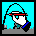Mathwright Books

This webpage makes available mathwright books I have developed. To use these activities, you must have Mathwright Player software. A free version, called the Mathwright Library Player, is available both here and at the New Mathwright Library and Cafe website. The software, and the exe files below, only work on computers using Microsoft Windows.

Each book available here is in its own self-extracting exe file, along with any supporting files needed with the book. If you click one of the book titles below, the corresponding exe file should be transferred to your machine. Save the file on your hard disk, and note the location because you will have to go to that folder to expand the file.

To expand one of these files, visit it in the file explorer and open it as you would any other file. The extraction process permits you to specify where to save the extracted files. If you simply click on unzip, a new folder will be created in the same location as the downloaded zip file.To run the activity, simply open the new folder, and then open the IMT file. The icon for an IMT file is shown at left.

Here is the list of mathwright books, with a brief description for each.

Mapping Geometry - (54 KB) Students enter a 2 by 2 matrix, and explore its behavior as a mapping from R2 to R2. There are several ways that they can enter subsets of the domain, and observe the images of these subsets.

Row Reduce - (52 KB) Students specify row operations by completing verbal descriptions, and see the operations carried out on a matrix. Any initial matrix from 2x2 up to 9x7 can be entered.

Marden's Theorem - (113 KB) This is an exploration of a theorem about the geometry of roots of complex polynomials and their derivatives. One version of the theorem says this: if the roots of a cubic polynomial are the vertices of a triangle in the complex plane, then the roots of the derivative are the foci of an ellipse inscribed in that triangle, and touching the sides at their midpoints. This file is a self extracting zip archive. So you should save it on your machine and then run it. It will create a directory with all the included files.

Areas and Riemann Sums - (73 KB) This activity gives students prolonged hands-on experience with the fundamental ideas of Riemann sums. The main activity is to construct a Riemann sum to estimate the area under a given curve. The student uses the mouse to select the endpoints of the interval, as well as the partition points. As each sub-interval is defined, a choice must be made by the student to establish a rectangular area: will the height of the rectangle be the function value at the left endpoint? The right endpoint? The midpoint? Or some other point selected by mouse click? Whatever choice is made, an animated display depicts the construction of the corresponding rectangle, and the area is automatically computed and added to a running total for the Riemann sum.

Epsilon and Delta - (79 KB) This activity concerns the epsilon-delta definition of limit, using a geometric formulation of the usual inequalities. For a specified epsilon, delta, a, and L, the graph of f stays in the box (centered at point (a,L) with width 2 delta and height 2 epsilon) if it extends from one side of the box to the other without going off the top or bottom. This formulation of 0 < | x - a | < delta => | f(x) - L| < epsilon is used by students to explore several examples.

Magnify - (97 KB) Two fundamental ideas of calculus, the limit and the derivative, are explored visually in an activity of successively zooming in on one point of a graph. If the graph eventually becomes a straight line, the derivative is the slope of that line. Students control the zooming in process and measure the slope geometrically. If the graph never becomes a straight line at any scale, the function is not differentiable (at the specified point). Similarly, the idea of the limit is explored by zooming in on the graph of a function over a punctured interval. The limit, if it exists, must be the unique point that can "fill in the gap" caused by the puncture in the domain.

Newton's Method - (42 KB) An animated illustration of the conventional picture of Newton's method. At each iteration, the student sees a ray trace the tangent line to the x axis, and determine a new value of x. The student defines the function and starting point for the iteration. In addition, a built-in example illustrates how sensitive an orbit can be to the initial value.

Polar Coordinate Grapher - (43 KB) A general purpose polar coordinates graphing environment featuring a variety of static and dynamic representations.

Parametric Equations - (128 KB) Mike Pepe's Parametric Equations Tutorial

Conics - (61 KB) Exploration of standard forms of equations for ellipses and hyperbolas

Star - (90 KB) An exploration of the geometry of stars. This is accessible to students at about any grade level, although there are some features that will not make sense to the early elementary grades. However, anyone who is familiar with measuring angles in degrees can use the activity to create beautiful stars. There is an included MS Word document (Star.doc) that serves as a user guide.

Elementary Models Collection - (390 KB) A suite of activities that can be used with the Elementary Mathematical Models course. Once you have expanded this archive, open the CONTENTS mathwright book for further information about each activity.